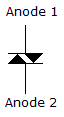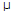# Electronic Devices - PNPN

### Exercise :: PNPN - General Questions

26.

This symbol is an example of a(n) ________.A. SCR B. SCS C. GTO D. DIAC

Explanation:

No answer description available for this question. Let us discuss.

27.

How many terminals does a silicon-controlled switch (SCS) device have?

 A. 2 B. 3 C. 4 D. 5

Explanation:

No answer description available for this question. Let us discuss.

28.

What is the typical value of the triggering anode gate for SCS devices?

 A. 1.5 mA B. 150 A C. 15 A D. 1 A

Explanation:

No answer description available for this question. Let us discuss.

29.

What is the typical range of turn-off times for SCRs?

 A. 5s to 30s B. 1s to 5s C. 0.1s to 1s D. 0.01s to 0.1s

Explanation:

No answer description available for this question. Let us discuss.

30.

Which of the following is (are) the advantages of the SCS over a corresponding SCR?

 A. Reduced turn-off time B. Increased control and triggering sensitivity C. More predictable firing situation D. All of the above